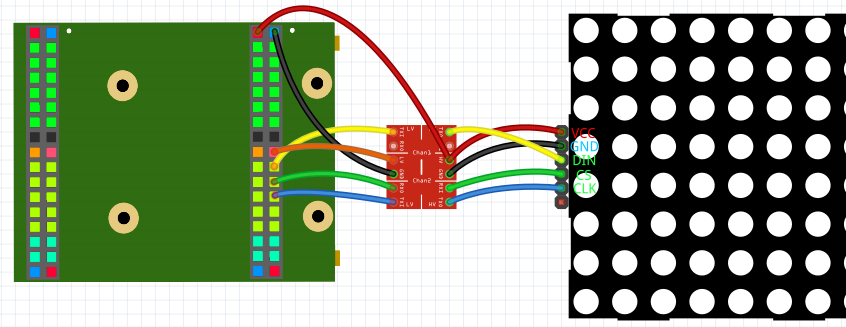# LED Matrix Sound Visualizer

## #What is an Sound Visualizer?

In this tutorial we want to use a microphone to record the ambient sound and then filter the different frequencies out of the sound. This is done by a so called "fast Fourier transform", that usualy is a complex operation, but quickly made by the FPGA. Finaly we want to display the result with an LED matrix.## #What you need

Important: This are only links for the german products.

### #1. Electronics

*This links are Affiliate Links. By purchasing with them you support us and our work, because we get a part of the revenue as commission. You still pay the same price.

## #The hardware

### #Soldering

Because there are no cheap level shifter boards pre-soldered, I would recommend learning some soldering. You could just plug the headers in the holes, but this doesn't ensure the connection and that the headers stay in place. Also soldering is pretty important for a lot of possible projects. Here is a cheap soldering station.

Otherwise here is a more expensive level shifter that comes pre-soldered.

Here you can see how to solder the headers to the level shifter.

### #Electronics

The led matrix* has to be connected with through a level shifter with the FPGA board, because the matrix works with 5V. The level shifter converts the 3.3V outputs of the FPGA to 5V outputs. Here you can see how to connect the matrix:5V and GND has to be conected with HV and GND of the level shifter and VCC and GND of the matrix. 3.3V has to be connected with LV of the level shifter. The 3 I/Os of the FPGA have to be connected with the LV I/Os of the level shifter. The LV I/Os then with the matrix.

To connect the microphone, you just have to connect 3.3V and GND of the microphone with 3.3V and GND of the FPGA and connect the rest of the pins (exept for SEL) with the FPGA I/Os.

## #The software

Create a new project and import the LED_Matrix library folder, the Spectrum_Analyzer library folder, the I2S_IN and the SPI library. Important: Make sure that you assign Brightness and Shutdown values or remove them from NewComponent. Also Config has to change from '0' to '1' when the matrix is connected. Now you can copy this example:

``Main(    --MEMS Microphone    LRCLK         : OUT STD_LOGIC;    BCLK          : OUT STD_LOGIC;    Data          : IN STD_LOGIC;    --LED Matrix    CS_O          : BUFFER STD_LOGIC;    CLK_O         : OUT STD_LOGIC;    DIN_O         : OUT STD_LOGIC;){    CONSTANT volume_divider : NATURAL := 8;     -- lower value = higehr volume    CONSTANT offset         : INTEGER := 6130;  -- Microphone value += offset, so median is 0        --Reads the Microphone library    SIGNAL I2S_Interface_IN_Data_L             : STD_LOGIC_VECTOR (18-1 downto 0);    SIGNAL I2S_Interface_IN_Data_R             : STD_LOGIC_VECTOR (18-1 downto 0);    SIGNAL I2S_Interface_IN_Ready              : STD_LOGIC;    NewComponent I2S_Interface_IN    (        CLK_Frequency      => 12000000,        Sampling_Frequency => 32000,        Data_Width         => 18,        Sample_Bits        => 32,        BCLK_Edge          => '1',                Reset              => '0',        LRCLK              => LRCLK,        BCLK               => BCLK,        Data               => Data,        Data_L             => I2S_Interface_IN_Data_L,        Data_R             => I2S_Interface_IN_Data_R,        Ready              => I2S_Interface_IN_Ready,    );    --Analyzes the micophone data    SIGNAL Spectrum_Analyzer_data_o    : Spectrum_Type;    SIGNAL Spectrum_Analyzer_New_Data  : STD_LOGIC;    NewComponent Spectrum_Analyzer    (        CLK_Frequency     => 12000000,        Sample_Frequency  => 10000,        Frequency_Numbers => 16,        Range_Multiplier  => 2,        adc_in            => resize((SIGNED(I2S_Interface_IN_Data_L)+offset)/volume_divider, 8),        New_Data          => Spectrum_Analyzer_New_Data,        data_o            => Spectrum_Analyzer_data_o,    );        --Create the LED Matix bitmap and initialize the matrix    Process ()    {        Thread        {            --Load settings (brightness and shutdown)            LED_Matrix_Config <= '0'; --Wait on start (not absolutely necessary)            Wait(10ms);            LED_Matrix_Config <= '1'; --Send settings            Step{ LED_Matrix_Config <= '0'; }            Wait(10ms);                        While(true)            {                For(i IN 0 to 31)                {                    If(Spectrum_Analyzer_data_o(i) > 127){LED_Matrix_Panel_Bitmap(i)(0) <= '1';}Else{LED_Matrix_Panel_Bitmap(i)(0) <= '0';}                    If(Spectrum_Analyzer_data_o(i) > 64) {LED_Matrix_Panel_Bitmap(i)(1) <= '1';}Else{LED_Matrix_Panel_Bitmap(i)(1) <= '0';}                    If(Spectrum_Analyzer_data_o(i) > 31) {LED_Matrix_Panel_Bitmap(i)(2) <= '1';}Else{LED_Matrix_Panel_Bitmap(i)(2) <= '0';}                    If(Spectrum_Analyzer_data_o(i) > 15) {LED_Matrix_Panel_Bitmap(i)(3) <= '1';}Else{LED_Matrix_Panel_Bitmap(i)(3) <= '0';}                    If(Spectrum_Analyzer_data_o(i) > 7)  {LED_Matrix_Panel_Bitmap(i)(4) <= '1';}Else{LED_Matrix_Panel_Bitmap(i)(4) <= '0';}                    If(Spectrum_Analyzer_data_o(i) > 3)  {LED_Matrix_Panel_Bitmap(i)(5) <= '1';}Else{LED_Matrix_Panel_Bitmap(i)(5) <= '0';}                    If(Spectrum_Analyzer_data_o(i) > 1)  {LED_Matrix_Panel_Bitmap(i)(6) <= '1';}Else{LED_Matrix_Panel_Bitmap(i)(6) <= '0';}                    If(Spectrum_Analyzer_data_o(i) > 0)  {LED_Matrix_Panel_Bitmap(i)(7) <= '1';}Else{LED_Matrix_Panel_Bitmap(i)(7) <= '0';}                }                                --Update image -> show already defined image                LED_Matrix_Update     <= '0';                Step { LED_Matrix_Update <= '1'; }                                --Wait for new data                While(Spectrum_Analyzer_New_Data = '0') {}            }        }    }    --Output the matrix data    SIGNAL LED_Matrix_Panel_Bitmap  : LED_Matrix_Array ((4*8)-1 downto 0);    SIGNAL LED_Matrix_Update        : STD_LOGIC;    SIGNAL LED_Matrix_Config        : STD_LOGIC;    NewComponent LED_Matrix    (        CLK_Frequency => 12000000,        Panels        => 4,        Rotate_seg    => '1',        Mirror        => '1',                Reset         => '0',        CS_O          => CS_O,        CLK_O         => CLK_O,        DIN_O         => DIN_O,        Panel_Bitmap  => LED_Matrix_Panel_Bitmap,        Update        => LED_Matrix_Update,        Config        => LED_Matrix_Config,    );}``

This example displays the analyzed frequencies with an 8x32 LED matrix.

Make sure that you choose the correct FPGA Pins that are connected with DIN, CL and CLK, that 3.3V is connected with the level shifter and that 5V is connected with the level shifter and the matrix.

You can find the full example here.

## #Conclusion

This is another example of a cool usefull project with an LED matrix. Here are some more:
1. Text/Image displayer
2. Clock with timer
3. Custom text displayer

## #Possible problems

### #Matrix is dark

1. Check if everything is connected properly (3.3V, 5V and GND connected? Does the level shifter have a connection? Correct Pins used?)
2. Is the program correct? (Shutdown => '0'? Does LED_Matrix_Config change from '0' to '1' when the matrix has power?)
3. Has the matrix a MAX7219 IC?
4. Check if the I2S_Interface_IN_Data_L data changes, so the microphone works properly. This code outputs the value with the matrix:
``While(true){    For(i IN 0 to 17)    {        LED_Matrix_Panel_Bitmap(i)(0) <= I2S_Interface_IN_Data_L(i);    }        --Update image -> show already defined image    LED_Matrix_Update     <= '0';    Step { LED_Matrix_Update <= '1'; }        Wait(50ms);}``

### #Last column always on

Try to play around with offset constant. The last column is the one for the lowest frequency and an offset is interpreted as a verry low frequency.

### #Not sensitive enought

You can set a lower value as volume_divider to increase sensitivity.

We hope you enjoyed the tutorial and feel free to check out

Last updated on by Leon Beier